Open in App
Not now

# Python | StackLayout in Kivy

• Difficulty Level : Basic
• Last Updated : 25 Aug, 2021

Kivy is a platform independent GUI tool in Python. As it can be run on Android, IOS, linux and Windows etc. It is basically used to develop the Android application, but it does not mean that it can not be used on Desktops applications.

### StackLayout:

To use StackLayout first import StackLayout by below command:

`from kivy.uix.stacklayout import StackLayout`

It is quite confusing to understand the difference between Stack and Boxlayout
StackLayout can organize widgets with more complexity than BoxLayout Boxlayout can organize widgets either in a vertical or horizontal way. But with the StackLayout, you can combine the orientations. There are 4 row-wise and 4 column wise orientations.

```More flexible that Boxlayout(1D)

StackLayout Orientation (2D):
- right to left or left to right
- top to bottom or bottom to top
- 'rl-bt', 'rl-tb', lr-bt', 'lr-tb'(Row wise)
- 'bt-rl', 'bt-lr', 'tb-rl', 'tb-lr'(Column wise)```

The four row-wise orientations and four column-wise orientation are shown in below pictures.

```Basic Approach to create Stack layout :

1) import kivy
2) import kivyApp
3) import Button
4) import Stacklayout
5) Set minimum version(optional)
6) create App class
7) return widget
8) Run an instance of the class```

Implementation of the Approach:

## Python3

 `# code to show how to use StackLayout`   `# import kivy module ` `import` `kivy `   `# this restricts the kivy version i.e ` `# below this kivy version you cannot ` `# use the app or software ` `kivy.require(``"1.9.1"``) ` `  `  `# base Class of your App inherits from the App class. ` `# app:always refers to the instance of your application ` `from` `kivy.app ``import` `App ` `  `  `# creates the button in kivy ` `# if not imported shows the error ` `from` `kivy.uix.button ``import` `Button`   `# The StackLayout arranges children vertically` `# or horizontally, as many as the layout can fit.` `from` `kivy.uix.stacklayout ``import` `StackLayout`     `# class in which we are creating StackLayout` `class` `StackLayoutApp(App): ` `      `  `    ``def` `build(``self``):`   `        ``# Different orientation` `        ``# ['lr-tb', 'tb-lr', 'rl-tb', 'tb-rl',` `           ``'lr-bt'``, ``'bt-lr'``, ``'rl-bt'``, ``'bt-rl'``]        ` `        ``SL ``=` `StackLayout(orientation ``=``'lr-tb'``)`   `        ``# Creating Multiple Buttons ` `        ``btn1 ``=` `Button(text ``=``"B1"``,` `                      ``font_size ``=` `20``,` `                      ``size_hint ``=``(.``2``, .``1``))` `        ``btn2 ``=` `Button(text ``=``"B2"``,` `                      ``font_size ``=` `20``,` `                      ``size_hint ``=``(.``2``, .``1``))` `        ``btn3 ``=` `Button(text ``=``"B3"``,` `                      ``font_size ``=` `20``,` `                      ``size_hint ``=``(.``2``, .``1``))` `        ``btn4 ``=` `Button(text ``=``"B4"``,` `                      ``font_size ``=` `20``,` `                      ``size_hint ``=``(.``2``, .``1``))` `        ``btn5 ``=` `Button(text ``=``"B5"``,` `                      ``font_size ``=` `20``,` `                      ``size_hint ``=``(.``2``, .``1``))` `        ``btn6 ``=` `Button(text ``=``"B6"``,` `                      ``font_size ``=` `20``,` `                      ``size_hint ``=``(.``2``, .``1``))` `        ``btn7 ``=` `Button(text ``=``"B7"``,` `                      ``font_size ``=` `20``,` `                      ``size_hint ``=``(.``2``, .``1``))` `        ``btn8 ``=` `Button(text ``=``"B8"``,` `                      ``font_size ``=` `20``,` `                      ``size_hint ``=``(.``2``, .``1``))` `        ``btn9 ``=` `Button(text ``=``"B9"``,` `                      ``font_size ``=` `20``,` `                      ``size_hint ``=``(.``2``, .``1``))` `        ``btn10 ``=` `Button(text ``=``"B10"``,` `                      ``font_size ``=` `20``,` `                      ``size_hint ``=``(.``2``, .``1``))`     `        ``# adding widgets` `        ``SL.add_widget(btn1)` `        ``SL.add_widget(btn2)` `        ``SL.add_widget(btn3)` `        ``SL.add_widget(btn4)` `        ``SL.add_widget(btn5)` `        ``SL.add_widget(btn6)` `        ``SL.add_widget(btn7)` `        ``SL.add_widget(btn8)` `        ``SL.add_widget(btn9)` `        ``SL.add_widget(btn10)`     `        ``# returning widgets` `        ``return` `SL`   `# run function runs the whole program ` `# i.e run() method which calls the ` `# target function passed to the constructor. ` `if` `__name__ ``=``=` `'__main__'``:` `    ``StackLayoutApp().run()`

Output: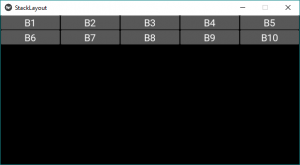This is for the orientation ‘lr-tb’. First the widgets are added left-to-right and then top-to-bottom.
Note: If want to change orientation, just change the orientation in line no 31 with any of the below orientations –

```For row wise orientation use:
-'lr-tb'
-'lr-bt'
-'rl-tb'
-'rl-bt'

For column wise orientation use:
-'tb-lr'
-'tb-rl'
-'bt-lr'
-'bt-rl'```

Below there are picture output all the orientations above –
For row wise orientation use:

`'lr-tb'`

Output:`'lr-bt'`

Output: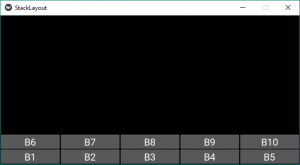`'rl-tb'`

Output: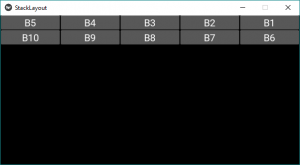`'rl-bt'`

Output: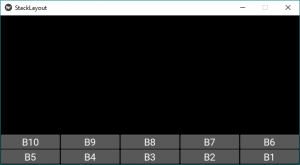For column wise orientation use:

`'tb-lr'`

Output: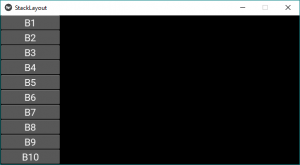`'tb-rl'`

Output: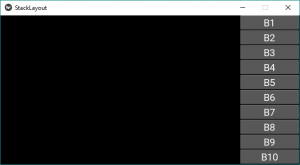`'bt-lr'`

Output: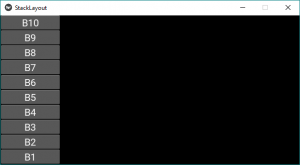`'bt-rl'`

Output: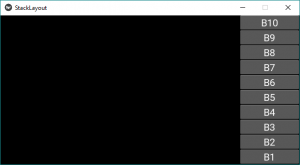My Personal Notes arrow_drop_up
Related Articles